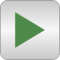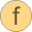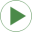# Examples

Examples to demonstrate the usage of package Modelica.Utilities# Package Contentscalculator Interpreter to evaluate simple expressions consisting of +, -, *, /, (), sin(), cos(), tan(), sqrt(), asin(), acos(), atan(), exp(), log(), piexpression Expression interpreter that returns with the position after the expression (expression may consist of +, -, *, /, (), sin(), cos(), tan(), sqrt(), asin(), acos(), atan(), exp(), log(), pi)readRealParameter Read the value of a Real parameter from filereadRealParameterModel Demonstrate usage of Examples.readRealParameter/.expressionWriteRealMatrixToFile Demonstrate usage of function Streams.writeRealMatrixReadRealMatrixFromFile Demonstrate usage of function Streams.readRealMatrix

# Information

This information is part of the Modelica Standard Library maintained by the Modelica Association.

This package contains quite involved examples that demonstrate how to use the functions of package Modelica.Utilities. In particular the following examples are present.

• Function calculator is an interpreter to evaluate expressions consisting of +,-,*,/,(),sin(), cos(), tan(), sqrt(), pi. For example: calculator("1.5*sin(pi/6)");

• Function expression is the basic function used in "calculator" to evaluate an expression. It is useful if the expression interpreter is used in a larger scan operation (such as readRealParameter below).

• Function readRealParameter reads the value of a parameter from file, given the file and the parameter name. The value on file is interpreted with the Examples.expression function and can therefore be an expression.

• Model readRealParameterModel is a test model to demonstrate the usage of "readRealParameter". The model contains 3 parameters that are read from file Examples_readRealParameters.txt.

• Model WriteRealMatrixToFile demonstrates the usage of function "Streams.writeReaMatrix" to store a matrix in various MATLAB MAT formats on file.